Need help? Email help@shout.education

# Buffer Solutions

This page describes simple acidic and alkaline buffer solutions and explains how they work.

## What is a Buffer Solution?

A buffer solution is one which resists changes in pH when small quantities of an acid or an alkali are added to it.

### Acidic Buffer Solutions

An acidic buffer solution is simply one which has a pH less than 7. Acidic buffer solutions are commonly made from a weak acid and one of its salts – often a sodium salt.

A common example would be a mixture of ethanoic acid and sodium ethanoate in solution. In this case, if the solution contained equal molar concentrations of both the acid and the salt, it would have a pH of 4.76. It wouldn't matter what the concentrations were, as long as they were the same.

You can change the pH of the buffer solution by changing the ratio of acid to salt, or by choosing a different acid and one of its salts.

Note: If you have a very weak acid and one of its salts, this can produce a buffer solution which is actually alkaline! I will comment briefly about this further down the page, but if you are doing buffer solutions at an introductory level this isn't likely to bother you.

If you need to know about calculations involving buffer solutions, you may be interested in my chemistry calculations book.

### Alkaline Buffer Solutions

An alkaline buffer solution has a pH greater than 7. Alkaline buffer solutions are commonly made from a weak base and one of its salts.

A frequently used example is a mixture of ammonia solution and ammonium chloride solution. If these were mixed in equal molar proportions, the solution would have a pH of 9.25. Again, it doesn't matter what concentrations you choose as long as they are the same.

## How Do Buffer Solutions Work?

A buffer solution has to contain things which will remove any hydrogen ions or hydroxide ions that you might add to it – otherwise the pH will change. Acidic and alkaline buffer solutions achieve this in different ways.

### Acidic Buffer Solutions

We'll take a mixture of ethanoic acid and sodium ethanoate as typical.

Ethanoic acid is a weak acid, and the position of this equilibrium will be well to the left:

\text{CH}_3\text{COOH}_{(aq)} \xrightleftharpoons{} \text{CH}_3\text{COO}^-_{(aq)} + \text{H}^+_{(aq)}

Adding sodium ethanoate to this adds lots of extra ethanoate ions. According to Le Chatelier's Principle, that will tip the position of the equilibrium even further to the left.

Note: If you don't understand Le Chatelier's Principle, follow this link before you go any further, and make sure that you understand about the effect of changes of concentration on the position of equilibrium.

The solution will therefore contain these important things:

• lots of un-ionised ethanoic acid;
• lots of ethanoate ions from the sodium ethanoate;
• enough hydrogen ions to make the solution acidic.

Other things (like water and sodium ions) which are present aren't important to the argument.

#### Adding an acid to this buffer solution

The buffer solution must remove most of the new hydrogen ions otherwise the pH would drop markedly.

Hydrogen ions combine with the ethanoate ions to make ethanoic acid. Although the reaction is reversible, since the ethanoic acid is a weak acid, most of the new hydrogen ions are removed in this way.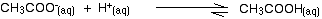Since most of the new hydrogen ions are removed, the pH won't change very much – but because of the equilibria involved, it will fall a little bit.

#### Adding an alkali to this buffer solution

Alkaline solutions contain hydroxide ions and the buffer solution removes most of these.

This time the situation is a bit more complicated because there are two processes which can remove hydroxide ions.

##### Removal by reacting with ethanoic acid

The most likely acidic substance which a hydroxide ion is going to collide with is an ethanoic acid molecule. They will react to form ethanoate ions and water.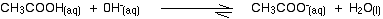Note: You might be surprised to find this written as a slightly reversible reaction. Because ethanoic acid is a weak acid, its conjugate base (the ethanoate ion) is fairly good at picking up hydrogen ions again to re-form the acid. It can get these from the water molecules. You may well find this reaction written as one-way, but to be fussy about it, it is actually reversible!

Because most of the new hydroxide ions are removed, the pH doesn't increase very much.

##### Removal of the hydroxide ions by reacting with hydrogen ions

Remember that there are some hydrogen ions present from the ionisation of the ethanoic acid.

\text{CH}_3\text{COOH}_{(aq)} \xrightleftharpoons{} \text{CH}_3\text{COO}^-_{(aq)} + \text{H}^+_{(aq)}

Hydroxide ions can combine with these to make water. As soon as this happens, the equilibrium tips to replace them. This keeps on happening until most of the hydroxide ions are removed.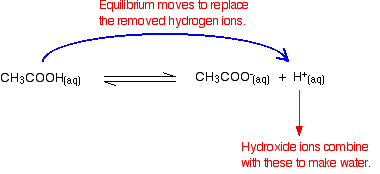Again, because you have equilibria involved, not all of the hydroxide ions are removed – just most of them. The water formed re-ionises to a very small extent to give a few hydrogen ions and hydroxide ions.

### Alkaline Buffer Solutions

We'll take a mixture of ammonia and ammonium chloride solutions as typical.

Ammonia is a weak base, and the position of this equilibrium will be well to the left:

\text{NH}_{3(aq)} + \text{H}_2\text{O}_{(l)} \xrightleftharpoons{} + {\text{NH}_4}^+_{(aq)} + {}^-\text{OH}_{(aq)}

Adding ammonium chloride to this adds lots of extra ammonium ions. According to Le Chatelier's Principle, that will tip the position of the equilibrium even further to the left.

The solution will therefore contain these important things:

• lots of unreacted ammonia;
• lots of ammonium ions from the ammonium chloride;
• enough hydroxide ions to make the solution alkaline.

Other things (like water and chloride ions) which are present aren't important to the argument.

#### Adding an acid to this buffer solution

There are two processes which can remove the hydrogen ions that you are adding.

##### Removal by reacting with ammonia

The most likely basic substance which a hydrogen ion is going to collide with is an ammonia molecule. They will react to form ammonium ions.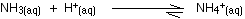Most, but not all, of the hydrogen ions will be removed. The ammonium ion is weakly acidic, and so some of the hydrogen ions will be released again.

Removal of the hydrogen ions by reacting with hydroxide ions

Remember that there are some hydroxide ions present from the reaction between the ammonia and the water.

\text{NH}_{3(aq)} + \text{H}_2\text{O}_{(l)} \xrightleftharpoons{} {\text{NH}_4}^+_{(aq)} + {}^-\text{OH}_{(aq)}

Hydrogen ions can combine with these hydroxide ions to make water. As soon as this happens, the equilibrium tips to replace the hydroxide ions. This keeps on happening until most of the hydrogen ions are removed.Again, because you have equilibria involved, not all of the hydrogen ions are removed – just most of them.

#### Adding an alkali to this buffer solution

The hydroxide ions from the alkali are removed by a simple reaction with ammonium ions.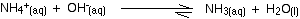Because the ammonia formed is a weak base, it can react with the water – and so the reaction is slightly reversible. That means that, again, most (but not all) of the the hydroxide ions are removed from the solution.

## Calculations involving buffer solutions

This is only a brief introduction. There are more examples, including several variations, over 10 pages in my chemistry calculations book.

### Acidic Buffer Solutions

This is easier to see with a specific example. Remember that an acid buffer can be made from a weak acid and one of its salts.

Let's suppose that you had a buffer solution containing 0.10 mol dm-3 of ethanoic acid and 0.20 mol dm-3 of sodium ethanoate. How do you calculate its pH?

In any solution containing a weak acid, there is an equilibrium between the un-ionised acid and its ions. So for ethanoic acid, you have the equilibrium:

\text{CH}_3\text{COOH}_{(aq)} \xrightleftharpoons{} \text{CH}_3\text{COO}^-_{(aq)} + \text{H}^+_{(aq)}

The presence of the ethanoate ions from the sodium ethanoate will have moved the equilibrium to the left, but the equilibrium still exists.

That means that you can write the equilibrium constant, Ka, for it:

K_a = \frac{[\text{CH}_3\text{COO}^-][\text{H}^+]}{[\text{CH}_3\text{COOH}]}

Where you have done calculations using this equation previously with a weak acid, you will have assumed that the concentrations of the hydrogen ions and ethanoate ions were the same. Every molecule of ethanoic acid that splits up gives one of each sort of ion.

That's no longer true for a buffer solution:

In a buffer solution, the concentration of A- and H+ are rarely, if ever, equal

If the equilibrium has been pushed even further to the left, the number of ethanoate ions coming from the ethanoic acid will be completely negligible compared to those from the sodium ethanoate.

We therefore assume that the ethanoate ion concentration is the same as the concentration of the sodium ethanoate – in this case, 0.20 mol dm-3.

In a weak acid calculation, we normally assume that so little of the acid has ionised that the concentration of the acid at equilibrium is the same as the concentration of the acid we used. That is even more true now that the equilibrium has been moved even further to the left.

So the assumptions we make for a buffer solution are:

K_a = \frac{\overbrace{[\text{CH}_3\text{COO}^-]}^{\clap{\text{\color{#467abf}{assume this is the same as the concentration of the sodium ethanoate}}}{}}[\text{H}^+]}{\underbrace{[\text{CH}_3\text{COOH}]}_{ \clap{ \text{ \color{#467abf}{assume this is the same as the concentration of the original acid} } }{} }}

Now, if we know the value for Ka, we can calculate the hydrogen ion concentration and therefore the pH.

Ka for ethanoic acid is 1.74 x 10-5 mol dm-3.

Remember that we want to calculate the pH of a buffer solution containing 0.10 mol dm-3 of ethanoic acid and 0.20 mol dm-3 of sodium ethanoate.

\begin{aligned} K_a &= \frac{[\text{CH}_3\text{COO}^-][\text{H}^+]}{[\text{CH}_3\text{COOH}]} \\ 1.74 \times 10^{-5} &= \frac{0.20 \times [\text{H}^+]}{0.10} \\ [\text{H}^+] &= 1.74 \times 10^{-5} \times \frac{0.20}{0.10} \\ {} &= 8.7 \times 10^{-6} \text{ mol} \text{ dm}^{-3} \end{aligned}

Then all you have to do is to find the pH using the expression pH = -log10 [H+]

You will still have the value for the hydrogen ion concentration on your calculator, so press the log button and ignore the negative sign (to allow for the minus sign in the pH expression).

You should get an answer of 5.1 to two significant figures. You can't be more accurate than this, because your concentrations were only given to two figures.

Note: I commented further up the page that if you had a very weak acid and one of its salts, the buffer solution formed could well be alkaline. An example is a mixture of HCN and NaCN.

HCN is a very weak acid with a Ka of 4.9 x 10-10 mol dm-3. If you had a solution containing an equal numbers of moles of HCN and NaCN, you could calculate (exactly as above) that this buffer solution would have a pH of 9.3.

This isn't something that you need to worry about. Just don't assume that every combination of weak acid and one of its salts will necessarily produce a buffer solution with a pH less than 7.

You could, of course, be asked to reverse this and calculate in what proportions you would have to mix ethanoic acid and sodium ethanoate to get a buffer solution of some desired pH. It is no more difficult than the calculation we have just looked at.

Suppose you wanted a buffer with a pH of 4.46. If you un-log this to find the hydrogen ion concentration you need, you will find it is 3.47 x 10-5 mol dm-3.

Feed that into the Ka expression.

\begin{aligned} K_a &= \frac{[\text{CH}_3\text{COO}^-][\text{H}^+]}{[\text{CH}_3\text{COOH}]} \\ 1.74 \times 10^{-5} &= \frac{[\text{CH}_3\text{COO}^-] \times 3.47 \times 10^{-5}}{[\text{CH}_3\text{COOH}]} \\ \frac{[\text{CH}_3\text{COO}^-]}{[\text{CH}_3\text{COOH}]} &= \frac{1.74 \times 10^{-5}}{3.47 \times 10^{-5}} \\ {} &= 0.50 \end{aligned}

All this means is that to get a solution of pH 4.46, the concentration of the ethanoate ions (from the sodium ethanoate) in the solution has to be 0.5 times that of the concentration of the acid. All that matters is that ratio.

In other words, the concentration of the ethanoate has to be half that of the ethanoic acid.

One way of getting this, for example, would be to mix together 10 cm3 of 1.0 mol dm-3 sodium ethanoate solution with 20 cm3 of 1.0 mol dm-3 ethanoic acid. Or 10 cm3 of 1.0 mol dm-3 sodium ethanoate solution with 10 cm3 of 2.0 mol dm-3 ethanoic acid. And there are all sorts of other possibilities.

Note: If your maths isn't very good, these examples can look a bit scary, but in fact they aren't. Go through the calculations line by line, and make sure that you can see exactly what is happening in each line – where the numbers are coming from, and why they are where they are. Then go away and practise similar questions.

If you are good at maths and can't see why anyone should think this is difficult, then feel very fortunate. Most people aren't so lucky!

### Alkaline Buffer Solutions

We are talking here about a mixture of a weak base and one of its salts – for example, a solution containing ammonia and ammonium chloride.

The modern, and easy, way of doing these calculations is to re-think them from the point of view of the ammonium ion rather than of the ammonia solution. Once you have taken this slightly different view-point, everything becomes much the same as before.

So how would you find the pH of a solution containing 0.100 mol dm-3 of ammonia and 0.0500 mol dm-3 of ammonium chloride?

The mixture will contain lots of unreacted ammonia molecules and lots of ammonium ions as the essential ingredients.

The ammonium ions are weakly acidic, and this equilibrium is set up whenever they are in solution in water:

{\text{NH}_4}^+_{(aq)} \xrightleftharpoons{} + \text{NH}_{3(aq)} + \text{H}^+_{(aq)}

You can write a Ka expression for the ammonium ion, and make the same sort of assumptions as we did in the previous case:

K_a = \frac{ \overbrace{ [\text{NH}_3] }^{ \clap{ \text{ \color{#467abf}{assume this is the same as provided concentration of ammonia} } }{} }[\text{H}^+] } {\underbrace{ [{\text{NH}_4}^+] }_{ \text{ \clap{ \color{#467abf}{assume this is the same as the concentration of the ammonium chloride} }{} } } }

The presence of the ammonia in the mixture forces the equilibrium far to the left. That means that you can assume that the ammonium ion concentration is what you started off with in the ammonium chloride, and that the ammonia concentration is all due to the added ammonia solution.

The value for Ka for the ammonium ion is 5.62 x 10-10 mol dm-3.

Remember that we want to calculate the pH of a buffer solution containing 0.100 mol dm-3 of ammonia and 0.0500 mol dm-3 of ammonium chloride.

Just put all these numbers in the Ka expression, and do the sum:

\begin{aligned} K_a &= \frac{ [\text{NH}_3][\text{H}^+] }{ [{\text{NH}_4}^+] } \\ 5.62 \times 10^{-10} &= \frac{ 0.100 \times [\text{H}^+] }{ 0.0500 } \\ [\text{H}^+] &= \frac{ 0.0500 }{ 0.100 } \times 5.62 \times 10^{-10} \\ {} &= 2.81 \times 10^{-10} \end{aligned}
\begin{aligned} pH &= {-}\log_{10}[\text{H}^+] \\ {} &= 9.55 \end{aligned}